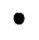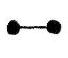# Spots Before My Eyes..

This problem concerns nodes and arcs.

 One nodeTwo nodes joined by 1 arcThree nodes joined by 3 arcs.No, we aren't asking for the number of arcs required to join a given-number of nodes one to another; that's been asked often enough before. Four nodes are joined by 6 arcs. I can draw this result asorIn the second diagram no arcs cross, while in the first we have produced an extra crossing-point.

So our first question is: for any given number of nodes, what is the minimum number of extra crossing-points we can have when each node is joined to every other;
and our second is: is there a formula connecting the number of nodes and the number of extra crossing-points?

I first suggested this topic to a class of 11 year olds - to be solved by trial and error.
By drawing, this class, and others, came up with :

Nodes (x)Extras (y)
40
51
63
79
819

And it isn't too difficult to find a formula to fit these results:
y = 2(x - 5)2 + 1;
which is fine as long as the y-values above are the real minima.

From the formula we can then predict the minima for other x-values. For x = 9, for example, the y-value is 33. By actual drawing the least number obtained by any class was 36; and then on the last day of term one of the Lower Sixth achieved y = 33 by drawing.

Perhaps others would like to try this problem to confirm, or disprove, my conjecture that the formula is correct.